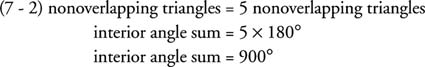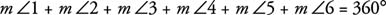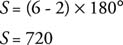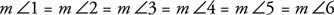## Angle Sum of Polygons

When you begin with a polygon with four or more sides and draw all the diagonals possible from one vertex, the polygon then is divided into several nonoverlapping triangles. Figure illustrates this division using a seven‐sided polygon. The interior angle sum of this polygon can now be found by multiplying the number of triangles by 180°. Upon investigating, it is found that the number of triangles is always two less than the number of sides. This fact is stated as a theorem.Figure 1 Triangulation of a seven‐sided polygon to find the interior angle sum.

Theorem 39: If a convex polygon has n sides, then its interior angle sum is given by the following equation: S = ( n −2) × 180°.

The polygon in Figure 1 has seven sides, so using Theorem 39 gives:An exterior angle of a polygon is formed by extending only one of its sides. The nonstraight angle adjacent to an interior angle is the exterior angle. Figure might suggest the following theorem:Figure 2 The (nonstraight) exterior angles of a polygon.

Theorem 40: If a polygon is convex, then the sum of the degree measures of the exterior angles, one at each vertex, is 360°.Example 1: Find the interior angle sum of a decagon.

A decagon has 10 sides, so:Example 2: Find the exterior angle sums, one exterior angle at each vertex, of a convex nonagon.

The sum of the exterior angles of any convex polygon is 360°.

Example 3: Find the measure of each interior angle of a regular hexagon (Figure 3).Figure 3 An interior angle of a regular hexagon.

Method 1: Because the polygon is regular, all interior angles are equal, so you only need to find the interior angle sum and divide by the number of angles.There are six angles, so 720 ÷ 6 = 120°.

Each interior angle of a regular hexagon has a measure of 120°.

Method 2: Because the polygon is regular and all its interior angles are equal, all its exterior angles are also equal. Look at Figure 2. This means thatBecause the sum of these angles will always be 360°, then each exterior angle would be 60° (360° ÷ 6 = 60°). If each exterior angle is 60°, then each interior angle is 120° (180° − 60° = 120°).